# Catch up on the latest AI articles# Let The Quadruped Robot Learn To Mimic Animals!

3 main points
✔️ Imitation learning using animal motion capture data
✔️ Achieve both robustness and adaptability to the environment
✔️ Reproduce multiple agile behaviors such as forward motion and turning on real machines

written by Xue Bin PengErwin CoumansTingnan ZhangTsang-Wei LeeJie TanSergey Levine
(Submitted on 2 Apr 2020 (v1), last revised 21 Jul 2020 (this version, v3))

Subjects: Robotics (cs.RO); Machine Learning (cs.LG)code：The images used in this article are from the paper, the introductory slides, or were created based on them.

## first of all

In this article, we introduce a paper that successfully implements quick movements in a quadruped robot in the real world, based on animal movements obtained by motion capture and animators' work.

The authors have also posted an explanation video. It's in English, but you can see how it works in the video, so please check it out too.

## introduction

Animals are capable of very agile movements. Although legged robots have the physical capability to perform agile motions, it is a time-consuming and labor-intensive task to create a controller for them. In this paper, we propose a framework to make the legged robot learn agile motions by imitating animal movements.

## technique

This method consists of the following three parts.

• motion retargeting
• Imitation of action

I would like to look at each of these in turn.

### motion retargeting

In this paper, we assume that we already have a working 3D model based on motion data captured from a real animal. Please note that we do not deal with the area of how to create the 3D model. In addition, a working 3D model does not have to be generated by motion capture and can be learned from a 3D model created by an animator.

When using animal motion data, it is necessary to correct the effects of skeletal differences. We use inverse kinematics to convert the original data into a form that conforms to the robot's skeleton.

The first step is to specify the corresponding points between the two models.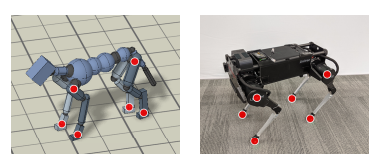We then define the joint angle sequence q0:T of the robot so that at each point the difference between the two models is reduced. This can be expressed as follows.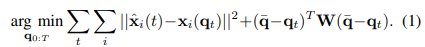Note that in the above equation, a regular additive term is added to prevent the robot from moving too far from the reference pose, and W is a diagonal matrix to represent the importance of each joint.

### Imitation of action

By the above procedure, we have obtained a sequence of joint angles q0:T as the teacher data. The next step is to train the system to take appropriate actions using this teacher data. In the mathematical expression, we learn the measure π( at|st, gt ), where gt is the information to be imitated, and gt= ( q^t+1, q^t+2, q^t+10, q^t+30 ). where q^ is the teacher data at the corresponding time. Since the sampling period is 30Hz, gt has information up to one second later. Also, the state st= ( qt-2:t, at-3:t-1 ).

The reward is expressed as a linear sum of multiple elements. The details are omitted, but it is calculated taking into account the deviation of position and velocity from the teacher data.

It will be a way to learn while being both robust and adaptive to the environment. This is the most important point in this paper.

### domain randomization

Domain randomization is a popular method for obtaining strategies that work in real-world simulations. It involves varying the dynamics of the environment during training to facilitate the acquisition of measures that work in several different environments.

The problems with this approach include the fact that not all strategies will work well in all environments, and that a strategy may work robustly in multiple environments in a simulation, but fail in the real world due to the effects of unmodeled elements.

To deal with the domain randomization problem mentioned earlier, we use a technique called Domain Adaptation. This method allows an agent to adapt to a new environment while learning how to behave robustly in multiple environments. Let's move on to the explanation of the specific method.

Let μ be the parameters of the environment. Specifically, it includes the mass and moment of inertia of the model and the delay time of the motor. During training, this μ is determined by sampling from a probability distribution p.

Then, through an encoder, μ is transformed into a latent representation z. RL is then formulated as finding the optimal action under a state s and a latent representation z of the environment. The idea is to find a policy π(a|s,z). That is to say, the behavior is not determined from the state alone as in ordinary RL, but it is determined by taking into account the information of the environment.

However, if we continue with the learning process, it will overly conform to a certain µ and lose robustness to the environment. To avoid this, we add a constraint to the encoder.

By setting an upper bound on the amount of mutual information between M(=μ) and z, we restrict the information that z has about the environment. In other words, the objective function can be expressed as follows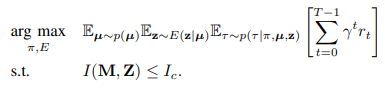Since it is difficult to calculate the mutual information I directly, we introduce a prior distribution of z, ρ(z), and substitute it in the upper bound of I. Note that ρ used in the experiment is the standard normal distribution.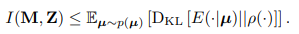By further relaxing the constraints and incorporating them in the equation, we finally obtain the following objective function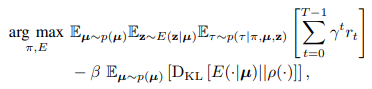We would like to turn to the interpretation of this equation (14). The first term is the same as in the usual RL, which is to find the pair of measures π and encoder E that maximizes the cumulative reward sum. The second term penalizes the information content of the encoder. This can be interpreted as a restriction on the environment that the agent can refer to by reducing the KL distance between the encoder and the prior distribution ρ. Also, β is a parameter that expresses the trade-off between robustness and adaptability of the learned strategy; a large β leads to learning robust but non-adaptive strategies. This corresponds to the case of domain randomization only; as β approaches 0, the system over-adapts to each environment and loses its ability to perform in the real environment.

## Deploying to the real world

Now that we've seen the procedure on the simulation, we'd like to look at deploying it in the real world. Of course, we cannot know z in the real world, so we need to guess it. We need to find z* such that the discounted reward sum is maximized, as in the following equation.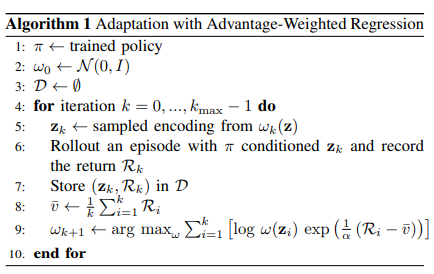To identify z*, we use a simple off-measure RL algorithm called advantage-weighted regression (AWR), which was proposed by the first author of this paper in 2019 and is used in this work to find a distribution that can better sample z distribution that can sample z better. The flow of the algorithm is described in the figure above, but we will look at each step in detail.

1. Prepare the learned measures.
2. Initially, we initialize the distribution Ω0 of z with a normal distribution.
3. Prepare a replay buffer to store z and the corresponding cumulative reward sum.
4. Repeat (5-9) up to k=0,1,... , kmax-1.
5. We will sample zk from Ωk.
6. We operate the actual machine using π and zk to obtain the cumulative reward sum Rk.
7. Store the pair of zk and Rk in the replay buffer D.
8. The average of the cumulative compensation sum to date is calculated as v-bar.
9. We create a new distribution by taking a weighted average over the log-likelihood of the previous distributions while increasing the weights of the distribution that samples z, which gives a large cumulative reward sum.

As for (9), it is possible to calculate it analytically because Ω is Gaussian, but it converges to a suboptimal solution prematurely, so it is calculated numerically using the steepest descent method for implementation.

## result

Using the above method, we have succeeded in making the Laikago robot learn various movements.